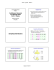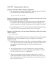# Small-Sample C.I.s for one- sample, two-sample and (matched) paired data

## Transcription

Small-Sample C.I.s for one- sample, two-sample and (matched) paired data
```Small-Sample C.I.s for onesample, two-sample and
(matched) paired data
Chapter 7: Estimation and
Statistical Intervals
2/20/12
Lecture 15- Xuanyao He
1
Review: In-Class Exercise
1.  What z critical value in the large-sample two-sided CI
for µ should be used to obtain each of the following
confidence levels?
a.  97%
b. 80%
c. 75%
2. Given these two-sided C.I.s for µ:
(6.71, 13.29) and (4.85, 15.15)
a.  What is the value of the sample mean?
b.  One of them is for confidence level = 90%, another
for 95%, which one has the 90% confidence level,
why?
2/20/12
Lecture 15- Xuanyao He
2
3. Suppose you want 90% of the area under the
sampling distribution of x to lie within ± 1 unit of a
population mean µ. Suppose the population
standard deviation is 3.
a) Find the minimum sample size n that satisfies this
requirement.
n = (1.645 *3/1 )^2 = 25, approximately.
b) What if the standard deviation is not given? We only
know the max. obs is 20, and min. is 10.
Use range/4 to estimate standard deviation,
and it’s (20-10)/4 = 2.5, therefore
n = (1.645 *2.5/1 )^2 = 17, approximately.
2/20/12
Lecture 15- Xuanyao He
3
7.4
Small-sample intervals Based on Normal
Population Distributions
X −µ
Z=
σ n
•  By replacing σ with s, we introduce a little extra
variability (due to random sampling, and s is a biased
point estimator of σ) by this substitution.
•  However, with a large n, the sampling distribution
still remains approximately normal.
•  Originally we started with
X −µ
–  Hence, Z =
s n
is still justified. As well as the
corresponding confidence interval, etc.
•  However, for small n (n< 25), this is no longer true!
2/20/12
Lecture 15- Xuanyao He
4
Effect of small samples using s
•  With small samples from the normal population
distribution, using s, is much more variable.
•  However, we can still standardize X using s, we
just need to get the “new” sampling distribution.
•  The standardized value will have a new
distribution, called the t (or Student’s t)
distribution:
X −µ
t=
s n
has the t distribution with n – 1 degrees of freedom.
2/20/12
Lecture 15- Xuanyao He
5
t distribution
•  There is a different t distribution for each
sample size (a.k.a. degrees of freedom or df )
•  The degrees of freedom for the t-statistic
“come” from the sample standard deviation s.
–  Recall: we had an “n – 1” in the calculation of s.
•  Good news
–  The density curve of a t distribution is:
•  Symmetric
•  Bell-shaped
•  Centered at 0
2/20/12
Lecture 15- Xuanyao He
6
t distribution
•  The higher the degrees of freedom (df) are, the narrower the spread of
the t distribution
n1 < n 2
df = n2
df = n1
0
•  As the df increase, the t density curve approaches the N(0, 1) curve
more closely
–  When df à ∞, t à z (standard normal).
•  Generally it is more spread than the normal, especially if the df are
small
2/20/12
Lecture 15- Xuanyao He
7
t distribution
2/20/12
Lecture 15- Xuanyao He
8
t distribution – table
•  On Page 566 of the textbook
•  For two-sided CI, locate the value of
central area and find the corresponding tcritical in the row of calculated d.f.
•  For one-sided CI, locate the relevant
cumulative area value and find
corresponding t-crit within the row of d.f.
2/20/12
Lecture 15- Xuanyao He
9
One sample t confidence interval
•  So the only thing that changes with the confidence intervals is we
substitute the z critical value by the t critical value.
•  One sample t confidence interval
s
X ± (t critical value)
n
•  t critical value from a t distribution with df = n – 1,
–  if that d.f. does not appear in the table on Page 566, pick up the closet df
to it;
–  if the df is some number in between two d.f.s in the table, pick up the
smaller one to be conservative, e.g. df = 35, then approach it by df = 30
in the table;
•  (And again, we could do upper and lower confidence bounds as well)
2/20/12
Lecture 15- Xuanyao He
10
Example 5
•
•
•
2/20/12
From a running production of corn soy blend we
take a sample to measure content of vitamin C. The
results are:
26 31 23 22 11 22 14 31
Find a 95% confidence interval for the content of
vitamin C in this production.
Notice: df = 8 – 1 = 7 here.
s
X ± (t critical value)
n
7.191
= 22.5 ± 2.365
×
≈ (16.49, 28.51)
Lecture 15- Xuanyao He
8
11
7.5
Two sample t confidence intervals
s12 s22
X 1 − X 2 ± (t crit )
+
n1 n2
•  The only difficulty here is the degrees of
freedom. We no longer have a simple n – 1.
df =
(s
2
1
(s
2
1
2
2
n1 + s n2
2
) (
2
2
2
)
2
)
n1
s n2
+
n1 − 1
n2 − 1
•  If the df is not an integer, round down to be
conservative (I.E. df = 9.86, use 9)
2/20/12
Lecture 15- Xuanyao He
12
Example 6
•
Metabolism rates of 12 random women and 7
random men were measured.
n1 = 12
x1 = 1235.1
s1 = 188.3
n2 = 7
x2 = 1600
s 2 = 189.2
•
Find a 95% confidence interval for the difference
in mean metabolism between men and women
•
Remember to interpret the interval!
2/20/12
Lecture 15- Xuanyao He
13
df =
(s
2
1
n1 + s n2 )
2
s
( 1 n1 )
n1 − 1
2
2
2
+
2
2
s
( 2 n2 )
2
= 12.6357 ≈ 12,
n2 − 1
So t-critical (for 95%)= 2.179, and 95% C.I. is
2
1
2
2
s
s
X 1 − X 2 ± (t crit )
+ = −364.9 ± 2.179 × 89.825
n1 n2
= [−560.629, −169.171]
2/20/12
Lecture 15- Xuanyao He
14
(Matched) Paired Data
•  Oftentimes, data is collected in pairs which creates the illusion
of two samples, although in reality there is really only one
sample.
•  Example—exams scores for STAT 350
Obs Exam 1 Exam 2
1
74
87
2
89
86
3
83
79
…
•  Why is this considered only one sample?
They are taken from the same group of individuals.
•  Other examples: pre and post results, married men vs.
women, measurements from twins
2/20/12
Lecture 15- Xuanyao He
15
Paired Data—treat like one sample
•  “Trick”—take the difference of the scores first, then
study the “differences” as a single sample
Obs
Exam 1
Exam 2
Difference
1
74
82
8
2
89
86
-3
3
83
79
-4
…
•  Find the mean of the differences.
•  Find the standard deviation of the differences.
•  What is the relevant confidence interval formula?
df?
2/20/12
Lecture 15- Xuanyao He
16
t C.I. for Paired Data
2/20/12
Lecture 15- Xuanyao He
17
Example 7
•  Suppose a sample of n students were given a diagnostic test
before and after completing a module. Here we have n1 = n2 =
10 students, the data is as follows:
•  Find a 95% confidence interval for the differences.
2/20/12
Lecture 15- Xuanyao He
18
•  Calculate the 90% C.I. for the difference
between post and pre scores.
2/20/12
Lecture 15- Xuanyao He
19
After Class…
•  Review sections 7.4 (till Pg 316) and 7.5
•  Read sections 8.1 and 8.2
–  Office hour: 3:30 – 6pm today
–  Handwritten Cheatsheet; SAT-calculator; student ID
•  Hw#5 is due by this Wed, 5pm.
•  Lab #3 – this Wed. Due by beginning of Fri’s class
2/20/12
Lecture 15- Xuanyao He
20
```

### SAMPLE MEANS 8/4/2014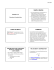### Activity - The IRIS Center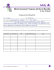### CALCULUS I Worksheet #69 1. Find all inflection points for f(x) = 2x3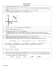### Chapter 23 Day 1 Objective: Construct confidence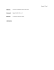### 1.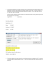### Math 3339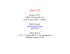### “CS 374” Spring 2015 — Homework 7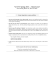### Her Home Housing Project - Backgrounder (Feb 2015 - For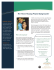### Chapter 7  μ Confidence Intervals and Sample Size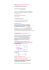### Blank Notes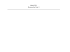### Typical Problem One Sample Estimation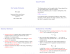### Lecture 3 Research Methods Lecture 10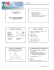### MODUL STATISTIKA UNTUK BISNIS DAN MANAJEMEN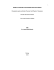### sections 7.1b-7.3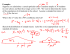### MIDTERM REVIEW SHEET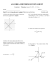### Confidence intervals for sample means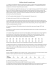### Chapter 14. Confidence Intervals: The Basics STAT 145 The usual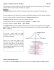### MATH 140 SEMESTER REVIEW PROBLEMS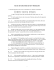### Exercises Test 3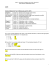### Document 6540260